﻿ Meet our 2018 Finalists and Judges

 Like Tweet
 /* styles */ August 16, 2018 Dear Readers, We are delighted to announce the 2018 Finalists for the Dayton Literary Peace Prize. This year’s finalists come from throughout the world—their countries of origin include the Czech Republic, Germany, Great Britain, South Korea, Palestine, Pakistan, Taiwan, and the U.S. Their books explore issues of immigration, occupation, ethnic wars, the power of education, justice and injustice, and African American history. We can learn much from their books and gain perspective on the current state of affairs at home and abroad, realizing that like the movement of the famed butterfly’s wing, the plight of people seemingly a world away has an effect that echoes throughout the globe. We invite you to meet the characters and people in these books whose lives connect with ours. You will also meet this year’s judges below, who are in the midst of reading these fine books and making the difficult decisions as to which books best fit our definitions of peace coupled with high literary quality. Information on the judging process can be found on our website.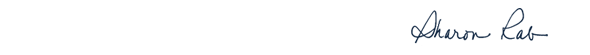table div table+table+table+table div table{width:100%;padding:0}table div table+table+table+table div table img{width:96.23%;padding:0;float:none}table div table+table+table+table div table td{width:100%;padding:0 1.88% 18px}/* styles */# Our Finalists - Fiction

 table div table+table+table+table+table+table div table{width:100%;padding:0}table div table+table+table+table+table+table div table img{width:96.23%;padding:0;float:none}table div table+table+table+table+table+table div table td{width:100%;padding:0 1.88% 18px}/* styles */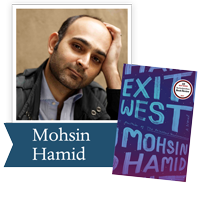Exit West by Mohsin Hamid (Riverhead): An astonishingly timely love story that brilliantly imagines the forces that transform ordinary people into refugees and the impossible choices that follow.

 table div table+table+table+table+table+table+table+table div table{width:100%;padding:0}table div table+table+table+table+table+table+table+table div table img{width:96.23%;padding:0;float:none}table div table+table+table+table+table+table+table+table div table td{width:100%;padding:0 1.88% 18px}/* styles */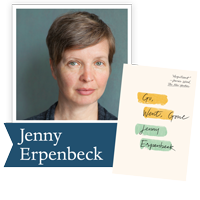Go, Went, Gone by Jenny Erpenbeck (New Directions): A scathing indictment of Western policy toward the European refugee crisis, but also a touching portrait of a man who finds he has more in common with the Africans than he realizes.

 table div table+table+table+table+table+table+table+table+table+table div table{width:100%;padding:0}table div table+table+table+table+table+table+table+table+table+table div table img{width:96.23%;padding:0;float:none}table div table+table+table+table+table+table+table+table+table+table div table td{width:100%;padding:0 1.88% 18px}/* styles */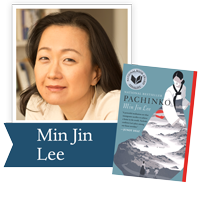Pachinko by Min Jin Lee (Grand Central): Four generations of a poor, proud immigrant Korean family fight to control their destinies, exiled from a homeland they never knew.

 table div table+table+table+table+table+table+table+table+table+table+table+table div table{width:100%;padding:0}table div table+table+table+table+table+table+table+table+table+table+table+table div table img{width:96.23%;padding:0;float:none}table div table+table+table+table+table+table+table+table+table+table+table+table div table td{width:100%;padding:0 1.88% 18px}/* styles */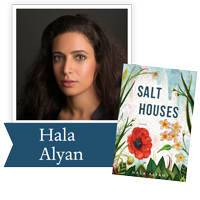Salt Houses by Hala Alyan (NMH): A heartbreaking story that follows three generations of a Palestinian family and asks us to confront that most devastating of all truths: you can’t go home again.

 table div table+table+table+table+table+table+table+table+table+table+table+table+table+table div table{width:100%;padding:0}table div table+table+table+table+table+table+table+table+table+table+table+table+table+table div table img{width:96.23%;padding:0;float:none}table div table+table+table+table+table+table+table+table+table+table+table+table+table+table div table td{width:100%;padding:0 1.88% 18px}/* styles */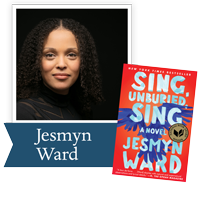Sing, Unburied, Sing by Jesmyn Ward (Scribner): Following a family making the trip from their Gulf Coast town to the Mississippi State Penitentiary, testing the strength of emotional bonds and the pull of our collective history.

 table div table+table+table+table+table+table+table+table+table+table+table+table+table+table+table+table div table{width:100%;padding:0}table div table+table+table+table+table+table+table+table+table+table+table+table+table+table+table+table div table img{width:96.23%;padding:0;float:none}table div table+table+table+table+table+table+table+table+table+table+table+table+table+table+table+table div table td{width:100%;padding:0 1.88% 18px}/* styles */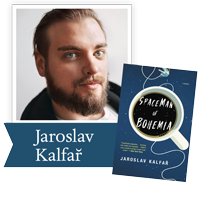Spaceman of Bohemia by Jaroslav Kalfař (Little, Brown): Raised in the Czech countryside by his doting grandparents, Jakub Procházka has risen from small-time scientist to become the country's first astronaut. A dangerous solo mission to Venus offers him the chance at heroism he's dreamt of and a way to atone for his father's sins as a Communist informer.

 table div table+table+table+table+table+table+table+table+table+table+table+table+table+table+table+table+table+table div table{width:100%;padding:0}table div table+table+table+table+table+table+table+table+table+table+table+table+table+table+table+table+table+table div table img{width:96.23%;padding:0;float:none}table div table+table+table+table+table+table+table+table+table+table+table+table+table+table+table+table+table+table div table td{width:100%;padding:0 1.88% 18px}/* styles */# Our Finalists - Nonfiction

 table div table+table+table+table+table+table+table+table+table+table+table+table+table+table+table+table+table+table+table+table div table{width:100%;padding:0}table div table+table+table+table+table+table+table+table+table+table+table+table+table+table+table+table+table+table+table+table div table img{width:96.23%;padding:0;float:none}table div table+table+table+table+table+table+table+table+table+table+table+table+table+table+table+table+table+table+table+table div table td{width:100%;padding:0 1.88% 18px}/* styles */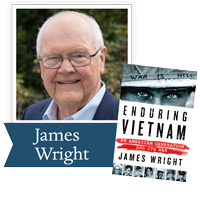Enduring Vietnam by James Wright (St. Martin’s Press): Recounts the experiences of the young Americans who fought in Vietnam and of families who grieved those who did not return.

 table div table+table+table+table+table+table+table+table+table+table+table+table+table+table+table+table+table+table+table+table+table+table div table{width:100%;padding:0}table div table+table+table+table+table+table+table+table+table+table+table+table+table+table+table+table+table+table+table+table+table+table div table img{width:96.23%;padding:0;float:none}table div table+table+table+table+table+table+table+table+table+table+table+table+table+table+table+table+table+table+table+table+table+table div table td{width:100%;padding:0 1.88% 18px}/* styles */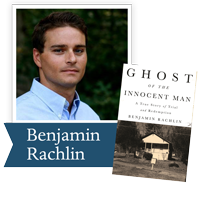Ghost of the Innocent Man by Benjamin Rachlin (Little, Brown): A gripping account of one man's long road to freedom that will forever change how we understand our criminal justice system through one of the most dramatic of those cases. It provides a picture of wrongful conviction and of the opportunity for meaningful reform.

 table div table+table+table+table+table+table+table+table+table+table+table+table+table+table+table+table+table+table+table+table+table+table+table+table div table{width:100%;padding:0}table div table+table+table+table+table+table+table+table+table+table+table+table+table+table+table+table+table+table+table+table+table+table+table+table div table img{width:96.23%;padding:0;float:none}table div table+table+table+table+table+table+table+table+table+table+table+table+table+table+table+table+table+table+table+table+table+table+table+table div table td{width:100%;padding:0 1.88% 18px}/* styles */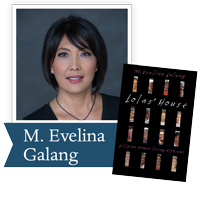Lolas’ House by M. Evelina Galang (Northwestern U. Press): Tells the stories, in unprecedented detail, of sixteen surviving Filipino “comfort women.” Not only a book of testimony and documentation, it is a book of witness, of survival, and of the female body.

 table div table+table+table+table+table+table+table+table+table+table+table+table+table+table+table+table+table+table+table+table+table+table+table+table+table+table div table{width:100%;padding:0}table div table+table+table+table+table+table+table+table+table+table+table+table+table+table+table+table+table+table+table+table+table+table+table+table+table+table div table img{width:96.23%;padding:0;float:none}table div table+table+table+table+table+table+table+table+table+table+table+table+table+table+table+table+table+table+table+table+table+table+table+table+table+table div table td{width:100%;padding:0 1.88% 18px}/* styles */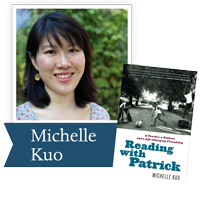Reading with Patrick by Michelle Kuo (Random House): In this stirring memoir, Kuo, the child of Taiwanese immigrants, shares the story of her complicated but rewarding mentorship of one student, Patrick Browning, and his remarkable literary and personal awakening.

 table div table+table+table+table+table+table+table+table+table+table+table+table+table+table+table+table+table+table+table+table+table+table+table+table+table+table+table+table div table{width:100%;padding:0}table div table+table+table+table+table+table+table+table+table+table+table+table+table+table+table+table+table+table+table+table+table+table+table+table+table+table+table+table div table img{width:96.23%;padding:0;float:none}table div table+table+table+table+table+table+table+table+table+table+table+table+table+table+table+table+table+table+table+table+table+table+table+table+table+table+table+table div table td{width:100%;padding:0 1.88% 18px}/* styles */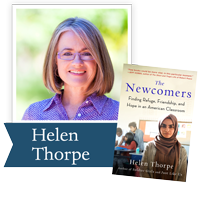The Newcomers by Helen Thorpe (Scribner): Helen Thorpe’s intensive, year-long reporting puts a human face on the faces of 22 newly-arrived teenagers taking a beginner-level English Language Acquisition class at South High School in Denver.

 table div table+table+table+table+table+table+table+table+table+table+table+table+table+table+table+table+table+table+table+table+table+table+table+table+table+table+table+table+table+table div table{width:100%;padding:0}table div table+table+table+table+table+table+table+table+table+table+table+table+table+table+table+table+table+table+table+table+table+table+table+table+table+table+table+table+table+table div table img{width:96.23%;padding:0;float:none}table div table+table+table+table+table+table+table+table+table+table+table+table+table+table+table+table+table+table+table+table+table+table+table+table+table+table+table+table+table+table div table td{width:100%;padding:0 1.88% 18px}/* styles */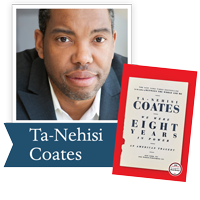We Were Eight Years in Power by Ta-Nehisi Coates (One World PRH): “Biting cultural and political analysis . . . reflects on race, Barack Obama’s presidency and its jarring aftermath, and his evolution as a writer in eight stunningly incisive essays. Kirkus Reviews (starred review)

 table div table+table+table+table+table+table+table+table+table+table+table+table+table+table+table+table+table+table+table+table+table+table+table+table+table+table+table+table+table+table+table+table div table{width:100%;padding:0}table div table+table+table+table+table+table+table+table+table+table+table+table+table+table+table+table+table+table+table+table+table+table+table+table+table+table+table+table+table+table+table+table div table img{width:96.23%;padding:0;float:none}table div table+table+table+table+table+table+table+table+table+table+table+table+table+table+table+table+table+table+table+table+table+table+table+table+table+table+table+table+table+table+table+table div table td{width:100%;padding:0 1.88% 18px}/* styles */# Meet our Judges

 table div table+table+table+table+table+table+table+table+table+table+table+table+table+table+table+table+table+table+table+table+table+table+table+table+table+table+table+table+table+table+table+table+table+table div table{width:100%;padding:0}table div table+table+table+table+table+table+table+table+table+table+table+table+table+table+table+table+table+table+table+table+table+table+table+table+table+table+table+table+table+table+table+table+table+table div table img{width:96.23%;padding:0;float:none}table div table+table+table+table+table+table+table+table+table+table+table+table+table+table+table+table+table+table+table+table+table+table+table+table+table+table+table+table+table+table+table+table+table+table div table td{width:100%;padding:0 1.88% 18px}/* styles */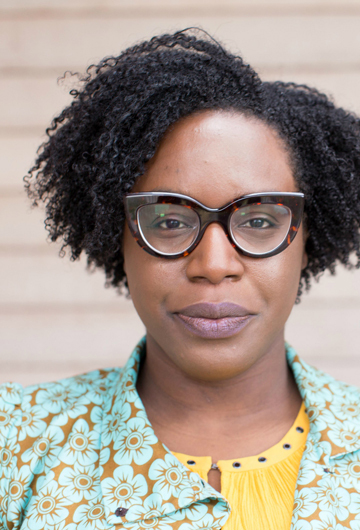Lesley Arimah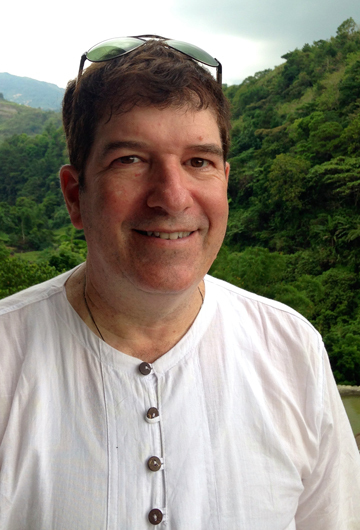Robin Hemley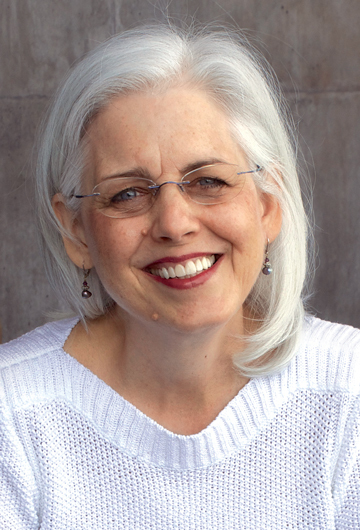Susan Southard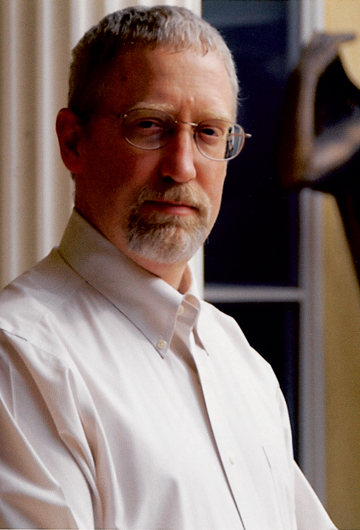Alan Taylor
 table div table+table+table+table+table+table+table+table+table+table+table+table+table+table+table+table+table+table+table+table+table+table+table+table+table+table+table+table+table+table+table+table+table+table+table+table div table{width:100%;padding:0}table div table+table+table+table+table+table+table+table+table+table+table+table+table+table+table+table+table+table+table+table+table+table+table+table+table+table+table+table+table+table+table+table+table+table+table+table div table img{width:96.23%;padding:0;float:none}table div table+table+table+table+table+table+table+table+table+table+table+table+table+table+table+table+table+table+table+table+table+table+table+table+table+table+table+table+table+table+table+table+table+table+table+table div table td{width:100%;padding:0 1.88% 18px}/* styles */## Fiction Judges

 table div table+table+table+table+table+table+table+table+table+table+table+table+table+table+table+table+table+table+table+table+table+table+table+table+table+table+table+table+table+table+table+table+table+table+table+table+table+table div table{width:100%;padding:0}table div table+table+table+table+table+table+table+table+table+table+table+table+table+table+table+table+table+table+table+table+table+table+table+table+table+table+table+table+table+table+table+table+table+table+table+table+table+table div table img{width:96.23%;padding:0;float:none}table div table+table+table+table+table+table+table+table+table+table+table+table+table+table+table+table+table+table+table+table+table+table+table+table+table+table+table+table+table+table+table+table+table+table+table+table+table+table div table td{width:100%;padding:0 1.88% 18px}/* styles *//* styles */ Lesley Nneka Arimah was born in the UK and grew up in Nigeria and wherever else her father was stationed for work. She has been a finalist for a National Magazine Award and the Caine Prize, and a winner of the African Commonwealth Short Story Prize, an O. Henry Award, and other honors. Her work has appeared in The New Yorker, Harper’s, GRANTA and has received support from The Elizabeth George Foundation, The Jerome Foundation, and MacDowell, among others. She was selected for the National Book Foundation’s 5 Under 35 and her debut collection WHAT IT MEANS WHEN A MAN FALLS FROM THE SKY won the 2017 Kirkus Prize. She lives in Minneapolis and is working on a novel about you.
 table div table+table+table+table+table+table+table+table+table+table+table+table+table+table+table+table+table+table+table+table+table+table+table+table+table+table+table+table+table+table+table+table+table+table+table+table+table+table+table+table div table{width:100%;padding:0}table div table+table+table+table+table+table+table+table+table+table+table+table+table+table+table+table+table+table+table+table+table+table+table+table+table+table+table+table+table+table+table+table+table+table+table+table+table+table+table+table div table img{width:96.23%;padding:0;float:none}table div table+table+table+table+table+table+table+table+table+table+table+table+table+table+table+table+table+table+table+table+table+table+table+table+table+table+table+table+table+table+table+table+table+table+table+table+table+table+table+table div table td{width:100%;padding:0 1.88% 18px}/* styles *//* styles */ Robin Hemley is the author of twelve books of nonfiction and fiction, and has won numerous awards for his writing, including a Guggenheim Fellowship, three Pushcart Prizes in both fiction and nonfiction, The Independent Press Book Award, an Editors Choice Award from The American Library Association, State Arts Council grants from Washington, North Carolina, and Illinois, The Ohioana Library Association Award, and fellowships from the Fine Arts Work Center in Provincetown, The MacDowell Colony, and Read more...
 table div table+table+table+table+table+table+table+table+table+table+table+table+table+table+table+table+table+table+table+table+table+table+table+table+table+table+table+table+table+table+table+table+table+table+table+table+table+table+table+table+table+table div table{width:100%;padding:0}table div table+table+table+table+table+table+table+table+table+table+table+table+table+table+table+table+table+table+table+table+table+table+table+table+table+table+table+table+table+table+table+table+table+table+table+table+table+table+table+table+table+table div table img{width:96.23%;padding:0;float:none}table div table+table+table+table+table+table+table+table+table+table+table+table+table+table+table+table+table+table+table+table+table+table+table+table+table+table+table+table+table+table+table+table+table+table+table+table+table+table+table+table+table+table div table td{width:100%;padding:0 1.88% 18px}/* styles */## Nonfiction Judges

 table div table+table+table+table+table+table+table+table+table+table+table+table+table+table+table+table+table+table+table+table+table+table+table+table+table+table+table+table+table+table+table+table+table+table+table+table+table+table+table+table+table+table+table+table div table{width:100%;padding:0}table div table+table+table+table+table+table+table+table+table+table+table+table+table+table+table+table+table+table+table+table+table+table+table+table+table+table+table+table+table+table+table+table+table+table+table+table+table+table+table+table+table+table+table+table div table img{width:96.23%;padding:0;float:none}table div table+table+table+table+table+table+table+table+table+table+table+table+table+table+table+table+table+table+table+table+table+table+table+table+table+table+table+table+table+table+table+table+table+table+table+table+table+table+table+table+table+table+table+table div table td{width:100%;padding:0 1.88% 18px}/* styles *//* styles */ Susan Southard holds an MFA in creative writing from Antioch University, Los Angeles, and was a nonfiction fellow at the Norman Mailer Center in Provincetown, Massachusetts. Her first book, Nagasaki: Life After Nuclear War (Viking, 2015) received the 2016 Dayton Literary Peace Prize in Nonfiction and the Lukas Book Prize, sponsored by the Columbia School of Journalism and Harvard University’s Nieman Foundation for Journalism. Nagasaki was also named a best book of the year by The Washington Post, The Economist, the American Library Association, and Kirkus Reviews. Read more...
 table div table+table+table+table+table+table+table+table+table+table+table+table+table+table+table+table+table+table+table+table+table+table+table+table+table+table+table+table+table+table+table+table+table+table+table+table+table+table+table+table+table+table+table+table+table+table div table{width:100%;padding:0}table div table+table+table+table+table+table+table+table+table+table+table+table+table+table+table+table+table+table+table+table+table+table+table+table+table+table+table+table+table+table+table+table+table+table+table+table+table+table+table+table+table+table+table+table+table+table div table img{width:96.23%;padding:0;float:none}table div table+table+table+table+table+table+table+table+table+table+table+table+table+table+table+table+table+table+table+table+table+table+table+table+table+table+table+table+table+table+table+table+table+table+table+table+table+table+table+table+table+table+table+table+table+table div table td{width:100%;padding:0 1.88% 18px}/* styles *//* styles */ Alan Taylor is an award-winning author and teacher. He taught in the history department at Boston University from 1987 to 1994. Since 1994, he has been a professor at the University of California at Davis. In 2002 he won the University of California at Davis Award for Teaching and Scholarly Achievement and the Phi Beta Kappa, Northern California Association, Teaching Excellence Award. Read more...
 table div table+table+table+table+table+table+table+table+table+table+table+table+table+table+table+table+table+table+table+table+table+table+table+table+table+table+table+table+table+table+table+table+table+table+table+table+table+table+table+table+table+table+table+table+table+table+table+table div table{width:100%;padding:0}table div table+table+table+table+table+table+table+table+table+table+table+table+table+table+table+table+table+table+table+table+table+table+table+table+table+table+table+table+table+table+table+table+table+table+table+table+table+table+table+table+table+table+table+table+table+table+table+table div table img{width:96.23%;padding:0;float:none}table div table+table+table+table+table+table+table+table+table+table+table+table+table+table+table+table+table+table+table+table+table+table+table+table+table+table+table+table+table+table+table+table+table+table+table+table+table+table+table+table+table+table+table+table+table+table+table+table div table td{width:100%;padding:0 1.88% 18px}/* styles */## Our 13th annual gala event on October 28 is sold out, but there are still opportunities to meet our authors on Sunday morning, October 28th, and through our Conversations with the Community at the Dayton Metro Library.

 table div table+table+table+table+table+table+table+table+table+table+table+table+table+table+table+table+table+table+table+table+table+table+table+table+table+table+table+table+table+table+table+table+table+table+table+table+table+table+table+table+table+table+table+table+table+table+table+table+table+table div table{width:100%;padding:0}table div table+table+table+table+table+table+table+table+table+table+table+table+table+table+table+table+table+table+table+table+table+table+table+table+table+table+table+table+table+table+table+table+table+table+table+table+table+table+table+table+table+table+table+table+table+table+table+table+table+table div table img{width:96.23%;padding:0;float:none}table div table+table+table+table+table+table+table+table+table+table+table+table+table+table+table+table+table+table+table+table+table+table+table+table+table+table+table+table+table+table+table+table+table+table+table+table+table+table+table+table+table+table+table+table+table+table+table+table+table+table div table td{width:100%;padding:0 1.88% 18px}/* styles */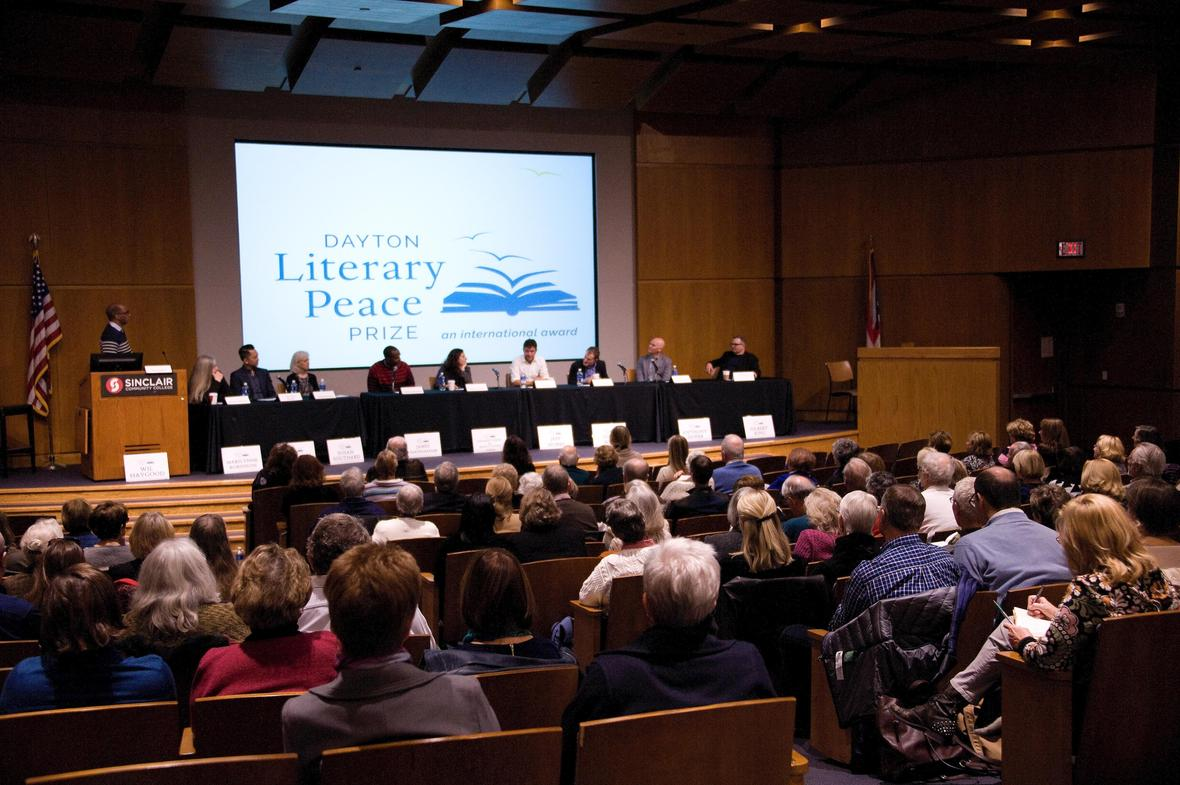## A Conversation with the 2018 Dayton Literary Peace Prize Winners

Limited seating: reservations accepted for the first 300

Sunday, October 28, 2018
9:00 AM Registration; 10:00 AM Program

Sinclair Community College, Ponitz Center, Building 12

Reservations are required. RSVP to Linda Harrison via e-mail by Wednesday, October 24, 2018. The \$15 donation is payable in cash or check to DLPP Foundation at the Registration Table. No credit cards. Limited seating; Reservations for first 300. Book signing after the program; Books will be available for purchase. Free underground parking is available under Building 12 off of W. 4th Street.

 table div table+table+table+table+table+table+table+table+table+table+table+table+table+table+table+table+table+table+table+table+table+table+table+table+table+table+table+table+table+table+table+table+table+table+table+table+table+table+table+table+table+table+table+table+table+table+table+table+table+table+table+table div table{width:100%;padding:0}table div table+table+table+table+table+table+table+table+table+table+table+table+table+table+table+table+table+table+table+table+table+table+table+table+table+table+table+table+table+table+table+table+table+table+table+table+table+table+table+table+table+table+table+table+table+table+table+table+table+table+table+table div table img{width:96.23%;padding:0;float:none}table div table+table+table+table+table+table+table+table+table+table+table+table+table+table+table+table+table+table+table+table+table+table+table+table+table+table+table+table+table+table+table+table+table+table+table+table+table+table+table+table+table+table+table+table+table+table+table+table+table+table+table+table div table td{width:100%;padding:0 1.88% 18px}/* styles *//* styles */ Visit the Dayton Literary Peace Prize Table at the 2018 Five Rivers Peacebuilder Experience Sunday, September 16, 1-5 pm at the Dayton Metro Library.
 table div table+table+table+table+table+table+table+table+table+table+table+table+table+table+table+table+table+table+table+table+table+table+table+table+table+table+table+table+table+table+table+table+table+table+table+table+table+table+table+table+table+table+table+table+table+table+table+table+table+table+table+table+table+table div table{width:100%;padding:0}table div table+table+table+table+table+table+table+table+table+table+table+table+table+table+table+table+table+table+table+table+table+table+table+table+table+table+table+table+table+table+table+table+table+table+table+table+table+table+table+table+table+table+table+table+table+table+table+table+table+table+table+table+table+table div table img{width:96.23%;padding:0;float:none}table div table+table+table+table+table+table+table+table+table+table+table+table+table+table+table+table+table+table+table+table+table+table+table+table+table+table+table+table+table+table+table+table+table+table+table+table+table+table+table+table+table+table+table+table+table+table+table+table+table+table+table+table+table+table div table td{width:100%;padding:0 1.88% 18px}/* styles */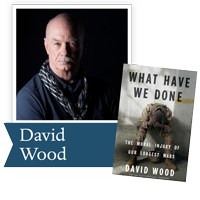## Join us for a DLPP Community Conversation at the Dayton Metro Library

8/30/2018 - 6:30 PM to 8:00 PM
Conference Room 1A, 1st Floor
Main Library, 215 E. 3rd St. Dayton, OH 45402

Dr. Jonathan Winkler, chair of the WSU Department of History and prominent military historian will lead the discussion of What Have We Done: The Moral Injury of Our Longest Wars, by David Wood. Community readers on the panel include Loghan Young, an Army Specialist and student Veteran at WSU. David Wood will skype with the audience to answer questions during the event.